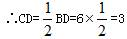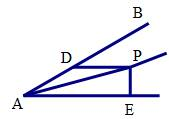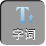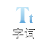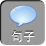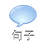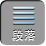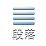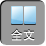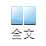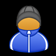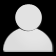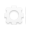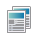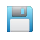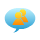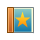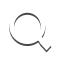-AA+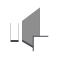∵⊿ ABC是等边三角形

AB= BC= AC,∠ BAC=∠ B=∠ C= 600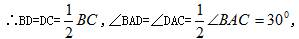RtABD中，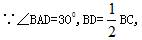AB= BC

BD=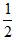AB.

1、已知⊿ ABCBA= BC，∠ ABC= 1200AB的垂直平分线交 AC于点 D，请问 ADDC的数量关系？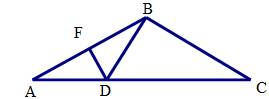BA= BC，∠ ABC= 1200

∴∠ A=∠ C= 300

AB的垂直平分线交 AC于点 D

∴∠ ABC=∠ A= 300

∴∠ DBC= 900

RtDBC中：

∵∠ C= 300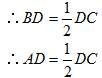2、（ 2007•天津）如图，△ ABC中，∠ C= 90°，∠ ABC= 60°， BD平分∠ ABC，若 AD= 6，则 CD为多少？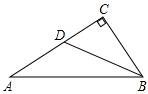∴∠ A= 30°，

BD平分∠ ABC

∴∠ CBD=∠ ABD=∠ A= 30°，